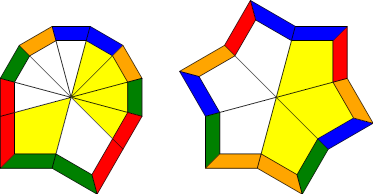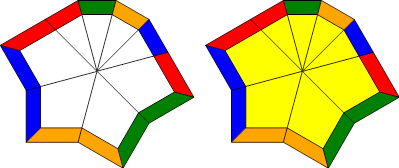# Cubeshape Parity

Cubeshape Parity, as the name suggests, involves fixing parity errors on the Square-1 during the cubeshape step. The main advantage to this method is that checking for parity occurs during inspection, which isn't factored into your final time. While the prospects of this method are groundbreaking, the method is still in development and is not currently being used in competitions by a lot of cubers, due to the amount of practice it takes to master this method.

Props to Matthew Sheerin for inventing his technique. The method is based off of this Speedsolving thread.

## First, What is Parity?

The term "parity" is often thrown around incorrectly, and the correct phrase that should be used is "parity error". Parity refers to the oddness/evenness of the number of swaps required to solve a puzzle. (For example, an Adj swap has odd parity, since it requires one swap, and an H-Perm is of even parity since it requires 2.) Notice that when you make a slice turn of the puzzle misaligned by 1,0, you swap 2 pairs of corners and 2 pairs of edges, making 4 swaps. Therefore, keeping the puzzle in cubeshape will maintain the puzzle in even parity.

Strange things happen when the Square-1 is not in the cubeshape, however. For example, setup the Square-1 with the moves /3,3/1,2/2,-2. Notice that by doing a slice move here, you swap 3 pairs of corners, resulting in 3 swaps and creating odd parity. Then, when you reverse the moves above, you will have a parity error, since you cannot return the puzzle to even parity by simply keeping it in cubeshape.

Colloquially, however, we simply use the word "parity" to refer to the fact that a puzzle has this parity error. In Cubeshape Parity (also known as CSP), we aim to fix parity errors during the cubeshape step.

## The Basic Idea

For every case of cubeshape, we assign what we call a reference scheme. This is a known position of the Square-1 in a particular cubeshape that makes it easier for us to determine the parity of the Square-1. Because parity relies on the number of swaps, essentially we will be counting how many swaps it takes to restore the puzzle to this reference scheme. Depending on whether we determine that there is even or odd parity, we will perform cubeshape in a particular way that restores the cubeshape and fixes even parity.

The best way to learn CSP is to provide examples. Consider the case 80/Star, with the following reference scheme: (images are viewed from the top of the puzzle)Pretend that this is the "solved" position of the 80/Star case; given a scramble in this case we need to count how many pair swaps it takes to return the puzzle to the reference scheme. If you know how to solve a Rubik's Cube blindfolded, it's the same principle; we count the cycles for where which every piece needs to go, except this time we don't care about the location of the pieces, we only care about whether the number is even or odd.

If you don't know what I'm talking about, here's a short example. Scramble your cube with the following scramble (I'm assuming you use white top, red front):

3,-1/1,-2/-1,-4/1,-2/2,0/
-3,-3/3,0/-1,-5/-4,-2/6,0

Starting with the YR edge, we find the place where it has to go in the reference scheme. In that place, there is a YB edge. We count "1" to represent we need one swap so far. Next, we see where the YB edge has to go, and we see that a WR edge is in its place. Then, we count "2" since now there are 2 swaps.

Now, since white-red goes back to where we started, we will have to start with a new cycle. and count the remaining number of swaps. We start with the WB edge, since the WG edge is already in its place. This has to go where the WO edge is, so we count "3". This returns the white-orange piece to its original position, meaning we have to start a new cycle. The only cycle that remains is between the YG and YO edges, which means we count up to "4". Thus, the edges require a total of 4 swaps to solve to the reference scheme.

We now do the same for the corners, without resetting the numbering. Without explanation, using the reference scheme we have the following cycles

WBO → YGO → WGR

WOG → YGR

YBR → WRB → YOB

meaning the corners require a total of 5 swaps. In total, we count 9 swaps, meaning the scramble has odd parity with respect to the reference scheme.

Now, for each cubeshape we require two ways of doing cubeshape that will either preserve or switch parity. It so happens that for the cases with a star shape, the two ways differ by turning the star layer by 2 units (I refer to this as a "star-shift"). This changes parity because it performs an equivalent of 5 swaps on the corners. If we end up counting an even number of swaps, we perform the cubeshape algorithm normally without a star-shift. If we count an odd amount of swaps, then we perform a star-shift and solve cubeshape normally. In our case, we have odd parity, so we would do

0,2/2,4/-2,-1/3,3/

where the red part indicates the star-shift that changes parity.

BE CAREFUL: for this particular case, we require the 8-group of edges to be in the back. If we solved cubeshape with it in the front, then our two ways of solving a parity error would be reversed, since this is equivalent to rotating the star layer by 6 units (3 swaps). Pay attention to these things when you learn cases.

## Another Example Trace

Here's another example trace, this time with the shape Scallop/Scallop. This has the reference scheme:We consider the scramble

-2,0/-1,-4/0,-3/0,-3/4,-2/2,-4/-5,0/-3,-3
/-5,-2/-4,0/4,0/-2,-2/4,6

The edges must cycle like so:

WO → YR → YG → WG → YB → WB → YO → WR

and the corners go as so:

WOG → YOB → WGR

YGO → YBR → WRB

We have 7 edge swaps and 4 corners swaps, resulting in 11 total swaps, which means we have odd parity. The odd parity algorithm for Scallop/Scallop is:

-2,2/2,-2/1,2/-3,-3/

The blue represents what I call a "slice-shift", which switches parity because it performs 3 corner swaps. It is also very prevalent in many CSP algorithms.

In this case, it does not matter whether the scallops were in the front or back; they achieve the same thing. For some cases the orientation will matter, and for other cases the orientation will not; you will need to keep them in mind when you learn CSP cases.

## A Few Things to Note

1. The star-shift and slice-shift will be very common in many algorithms, but that does not mean they are always in the odd parity algorithms; it depends on the specific case.

2. It does not matter what color scheme you choose to use, as long as you keep it consistent between white and yellow. I simply use this one because I'm used to it and have been using it ever since. For edges, going clockwise,

red, green, orange, blue

usually starting with white on top and yellow on bottom. For corners, it is the same thing by considering the sticker to the right.

3. For mirrors (when the layers are switched), you can either perform a z2 if the middle layer is flipped, or you can trace with white/yellow inverted.

## List of Cases

I would list all the cases out here, but there are just too many of them. Luckily, thanks to the efforts of Tommy Szeliga and Rowe Hessler, they have created a Google Doc with all the cases you need to know for CSP. You can find this doc here.

## Video Series

Alongside with this doc, I am also creating a video series detailing all the CSP cases. You can find a link to the playlist here.

## Concluding Remarks

CSP is very difficult to begin with, but once you get the hang of it you will start seeing massive improvements to your times. If you have any questions/needed clarifications about this guide, don't hesitate to contact me through my Facebook page or YouTube channel. Happy Squaning!